# How to use the DB Function in Excel

DB function is fixed declining depreciation is the way of calculating the different depreciation of any asset for each period.
The DB function returns depreciation amount for the input data for the specific product.
Syntax:

=DB (cost, salvage, life, period, [month])

Cost: Cost price of the asset
Salvage: remaining price of the asset after its lifetime period
Life: period after which cost price becomes salvage price
Period: specific period for which we need to calculate depreciation.
Month: [optional] specify month in a year. Default is 12 a year.

Let’s understand this function using it in an example.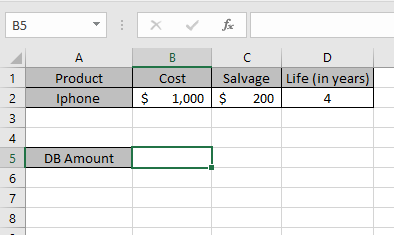Here we took a product as iPhone. The cost price & salvage price of the latest iPhone is shown in the snapshot below.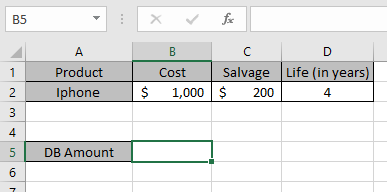Now we need to calculate the depreciation amount for the Iphone for first year.
Use the formula to calculate depreciation for first year:

=DB(B2,C2,D2,1)

B2 : Cost price of the asset
C2: the remaining price of the asset after a period
D2 : 4 years after cost price becomes salvage price
1 : first year to calculate depreciation amount.
Month : taken as default to 12 a year.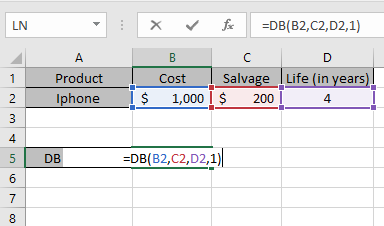The values to the formula are taken as cell reference.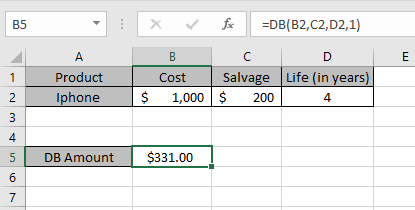As you can see from the above returned value, first year \$ 331 will depreciated from the asset cost value.
Now we will calculate the depreciation amount for each year separately.
Use the formula to calculate depreciation for second year:

=DB(B2,C2,D2,2)

B2 : Cost price of the asset
C2 : remaining price of the asset after a period
D2 : 4 years after cost price becomes salvage price
2 : first year to calculate depreciation amount.
Month : taken as default to 12 a year.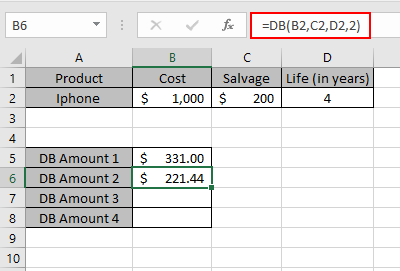Similarly calculate the depreciation for Third & Fourth year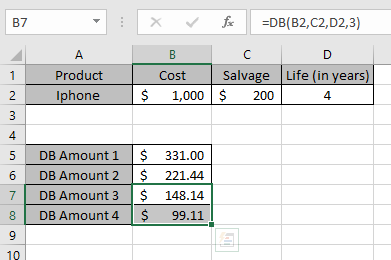So, we got different depreciation for each period.

 Year Depreciation Salvage value 0 0 \$ 1000 1 \$ 331.00 \$ 669.00 2 \$ 221.44 \$ 448.56 3 \$ 148.14 \$ 299.42 4 \$ 99.11 \$ 200.31

This is the drop depreciation table for the asset.
DB function shows that depreciation reduces as year passes away.
You can see from the above table that depreciation amount is deducted from the salvage amount for every year up till life of an asset.
Note:

1. The function generates #VALUE! Error if arguments to the function is non - numeric
2. The function generates #NUM! Error if:
• The value of cost and salvage is less than 0.
• The value of life of asset is less than or equals to zero.
• The period argument is less than or equals to 0 or greater than life period.
• The month argument is less than or equals to 0 or greater than 12.

Hope you understood how to use DB function and referring cell in Excel. Explore more articles on Excel  financial functions here. Please feel free to state your query or feedback for the above article.

Related Articles:

How to use the VDB Function in Excel

How to use the DDB Function in Excel

How to use the SYD Function in Excel

Popular Articles:

50 Excel Shortcuts to Increase Your Productivity

How to use the VLOOKUP Function in Excel

How to use the COUNTIF in Excel 2016

How to use the SUMIF Function in Excel

Terms and Conditions of use

The applications/code on this site are distributed as is and without warranties or liability. In no event shall the owner of the copyrights, or the authors of the applications/code be liable for any loss of profit, any problems or any damage resulting from the use or evaluation of the applications/code.